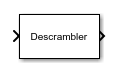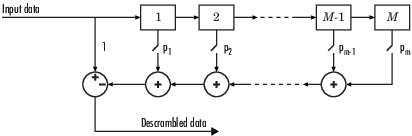# Descrambler

Descramble input signal

•Libraries:
Communications Toolbox / Sequence Operations

## Description

The Descrambler block applies multiplicative descrambling to input data. It performs the inverse operation of the Scrambler block used in the transmitter.

This schematic shows the multiplicative descrambler operation. The adders and subtracter operate modulo N, where N is the value specified by the `Calculation base` parameter.At each time step, the input causes the contents of the registers to shift sequentially. Using the `Scramble polynomial` parameter, you specify the on or off state for each switch in the descrambler. To make the Descrambler block reverse the operation of the Scrambler block, use the same parameter settings in both blocks. If there is no signal delay between the scrambler and the descrambler, then the Initial states in the two blocks must be the same.

To achieve repeatable initial descrambler conditions, you can use one of these optional input ports:

This block can accept input sequences that vary in length during simulation. For more information about sequences that vary in length, see Variable-Size Signal Basics (Simulink).

Note

To apply additive descrambling to input data, you can use the PN Sequence Generator block and the Logical Operator (Simulink) block configured as an XOR logical operator. For an example, see Additive Scrambling of Input Data in Simulink.

## Ports

### Input

expand all

Input data signal, specified as an NS-by-1 vector. NS represents the number of samples in the input signal. The input values must be integers from `0` to ```Calculation base````1`.

Data Types: `double` | `Boolean` | `int8` | `int16` | `int32` | `uint8` | `uint16` | `uint32`

Reset scrambler, specified as a scalar. The scrambler is reset if a nonzero input is applied to the port.

#### Dependencies

To enable this port, set ```Initial states source``` to ```Dialog Parameter``` and select `Reset on nonzero input via port`.

Initial states of the descrambler registers when the simulation starts, specified as a nonnegative integer vector. The length of ISt must equal the order of the ```Scramble polynomial``` parameter. The vector element values must be integers from `0` to ```Calculation base````1`.

#### Dependencies

To enable this port, set ```Initial states source``` to ```Input port```.

### Output

expand all

Output descrambled data, returned as an NS-by-1 vector. NS equals the number of samples in the input signal.

Data Types: `double`

## Parameters

expand all

Calculation base used in the descrambler for modulo operations, specified as a nonnegative integer. The input and output of this block are integers from `0` to Calculation base`1`.

Polynomial that defines the connections in the descrambler, specified as a character vector, integer vector, or binary vector. The Scramble polynomial parameter defines if each switch in the descrambler is on or off. Specify the polynomial as:

• A character vector, such as ```'1 + x^-6 + x^-8'```. For more details on specifying polynomials in this way, see Representation of Polynomials in Communications Toolbox.

• An integer vector, such as `[0 -6 -8]`, listing the descrambler coefficients in order of descending powers of x-1, where p(x-1) = 1 + p1x-1 + p2x-2 + ...

• A binary vector, such as ```[1 0 0 0 0 0 1 0 1]```, listing the powers of x that appear in the polynomial that has a coefficient of 1. In this case, the order of the descramble polynomial is one less than the binary vector length.

Example: `'1 + x^-6 + x^-8'`, ```[0 -6 -8]```, and `[1 0 0 0 0 0 1 0 1]` all represent this polynomial:

p(x-1) = 1 + x-6 + x-8

Initial states of descrambler registers when the simulation starts, specified as a nonnegative integer vector. The length of Initial states must equal the order of the ```Scramble polynomial``` parameter. The vector element values must be integers from `0` to ```Calculation base````1`.

#### Dependencies

This parameter is available when ```Initial states source``` is set to ```Dialog Parameter```.

Select this parameter to reset the Descrambler block via input port `Rst`.

#### Dependencies

This parameter is available when ```Initial states source``` is set to ```Dialog Parameter```.

## Block Characteristics

 Data Types `Boolean` | `double` | `integer` Multidimensional Signals `no` Variable-Size Signals `no`

## Version History

Introduced before R2006a